# NEWTON LAW OF MOTION

INERTIA

Inertia is defined as the ability of a body at rest to resist motion or a body in motion to continue moving in a straight line when abruptly stopped.
Newton’s first law of motion
It sometimes called “the law of Inertia
It states that “Everybody continues its state of rest or uniform motion in a straight line if there is no external force acting on it”
Momentum
A body is said to be in motion if it changes its position with time and when it has velocity
A body with zero velocity therefore it is not in motion and hence it is at rest
The motion of a body can be measured by multiplying out its mass ‘m’ and its velocity ‘v’ the product M.V is known as the linear momentum of a body
Linear momentum = Mass X Velocity
= kg X m/s
= kgm/s
... The S.I unit of momentum is kgm/s
Newton’s second law of motion
It states that the “rate of change of linear momentum of a body is directly proportional to the applied force and takes place in the direction of the force”
• Suppose force F acts on a body of mass ‘m’ for time t. This force causes the velocity of the body to change from initial velocity ‘u’ to find velocity ‘v’ in that interval t
•The change in momentum will then be Mv – Mu (kgm/s)
•The rate of change of momentum is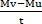, by Newton’s second law of motion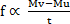.  Hence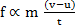.  But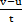= a (acceleration of a body). FMa
•If a constant of proportionality K is introduced in the above relation, then F = kMa. This equation can be used to define unit of force. If m = 1kg and a = 1m/s2, then the unit of force is chosen in such a way that when F = 1 the constant K = 1, hence F = Ma
•If a mass of 1kg is accelerating with 1m/s2, then a force 1N is said to be acting on the body. Therefore a force F of 1N can be defined as the force which when acting on the body of mass 1kg produces an acceleration of 1m/s2, That  is 1N = 1Kgm/s2 then constant of proportionality K will be equal to one.
Thus;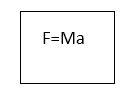MASS AND WEIGHT
In the earth gravitational field, the acceleration due to gravity is given by the symbol 'g'.
The gravitational force therefore which act on a body of mass ‘m’ is equal to mg. This is what is known as the weight of the body. This force tends to pull the body towards center of the earth.
Newton’s third law of motion
• It states that: “In every action there is an equal and opposite reaction”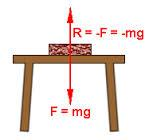•If a body of a mass ‘m’ is placed on a table as shown above, the body presses on the surface of the table with a force mg, the table supports the body with an upward force. The force which is the weight of the body is called the action of force while the upward force provided by the table is known as the reaction force. By Newton’s third law of motion the body does not move upwards or downwards.
Impulse of a force
•When a constant force F acts on a body of mass ‘m’, it produces an acceleration such that F = Ma. If the velocity of the body changes from U to V in time t, then;
a =From F = Ma
F =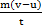F =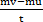Ft = Mv – Mu
The quantity Ft is called the impulse of a force. The S.I unit of impulse of a force is the Newton second (Ns)
EXAMPLES
1.      Find the linear momentum of a body of mass 5kg moving with the velocity of 2m/s.
Solution
Data given
Mass = 5kg
Velocity = 2m/s
Linear momentum = mass X velocity
= 5kg X 2m/s
= 10Kgm/s
2.      A football was kicked into hands of a goal keeper at 4m/s. The goal keeper stopped the ball in 2 seconds. If the mass of a ball is 0.5kg, calculate the average force exerted on the goal keeper

Solution

Data given

U = 4m/s
T = 2s
Mass = 0.5kg
V = 0m/s
F = Ma
F =F =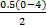F =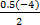F = -1N
The average force exerted by the goal keeper is 1N
3.      What force is required to give a mass of 0.2kg an acceleration of 0.5m/s2?

Solution

Data given

Mass = 0.2kg
Acceleration (a) = 0.5m/s2
From F = acceleration X mass
F = 0.2kg X 0.5m/s2
F = 0.1N
4.      What acceleration will be given to a body of mass 6kg by a force of 15N?

Solution

Data given

Mass = 6kg
Force = 15N
Acceleration (a) =?
From force = mass x acceleration
Acceleration =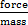Acceleration =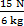A = 2.5m/s2
5.      What mass will be given an acceleration of 5m/s2 by a force 2N?

Solution
A = 5m/s2
F = 2N
M =?
Force =mass x acceleration
Mass =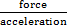Mass =Mass = 0.4kg
CONSERVATION OF A LINEAR MOMENTUM
Consider the case of firing a gun, as the bullet leaves the gun (reaction), the one holding it feels a backward force (reaction from the butt of the gun)
According to Newton’s third law of motion, these two forces are equal and opposite. Since these two forces act at the same time, the impulses (i.e. change in momentum) produced must be equal in magnitude and opposite in direction. The sum of the two momentum is equal to zero.
This implies that momentum cannot be produced some where without producing an equal and opposite momentum somewhere else. This is the law of conservation of linear momentum which states that “When two or more bodies act upon each other, their total momentum remains constant provided no external forces are acting”
Consider the collision of two balls moving in a straight line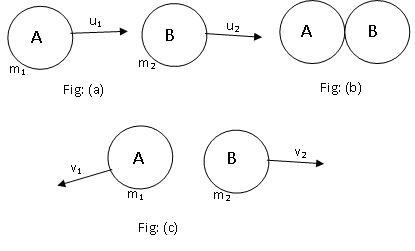The balls have the masses M1 and M2 and they are approaching each other with velocity U1 and U2 fig (a)
The balls have the velocities V1 and V2 after collision fig (b)
Let F1 and F2 be the forces acting on M1 and M2 during collision.
By Newton’s third law of motion the forces are equal and opposite since the two forces act during the same time t, the impulses produced are therefore equal and opposite
... F1t = -F2t
But F1t = M1V1 – M1U1
F2t = M2V2 – M2U2
From;
F1t = -F2t
M1V1 – M1U1 = -M2V2 + M2U2
M1V1 + M2V2 =M1U1 + M2U2
M1U1 + M2U2 = M1V1 + M2V2
This shows that the total momentum before collision is equals to the total momentum after collision.
Question
1.       A body of mass 8kg moving with velocity of 20m/s collides with another body of mass 4kg moving with a velocity of 10m/s in the same direction. The velocity of 8kg body is reduced to 15m/s after collision. Calculate the final velocity of the 4kg body

Solution

Data given
M1 = 8kg
U1 = 20m/s
M2 = 4kg
U2 = 10m/s
V1 = 15m/s
V2 =?
Apply
M1U1 + M2U2 = M1V1 + M2V2
8 x20 + 4 x 10 = 8 x 15 + 4 x V2
160 + 40 = 120 + 4V2
200 = 120 + 4V2
200 – 120 = 4V2
80 = 4V2
V2 = 20m/s
... The final velocity is 20m/s
EXERCISE
1.      Define the term momentum.
A car of mass 500kg is moving in a straight line with a velocity of 90km/h. calculate the linear momentum of the car
Solution
Momentum - is the product of mass and velocity
Data given
Mass = 500kg
Velocity = 90km/h
Linear momentum = mass X velocity
= Kg X m/s
Velocity = 90km/h
Convert km into m
Velocity = 90m/h =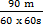Velocity = 25m/s
Momentum = 500kg X 25m/s
... Momentum is 12500kgm/s
2.      After striking its target a bullet of mass 50g is brought to rest in 2 seconds by a force of 300N. Calculate the velocity of a bullet before striking a target
Solution
Data given
Mass = 50g
Time = 2s
Force = 300N
Velocity =?
Convert g into kg
50g = 0.05kg
F =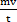300 =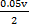V =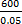V = 12000m/s
3.      A man of mass 80kg jumps off a trolley of mass 160kg. If the initial speed of the man is 8m/s, at what initial speed will the trolley move?
Solution
Data given
Velocity = 0m/s
Mass (M1) = 80kg
Speed (U1) = 8m/s
Mass (M2) = 160kg
M1U1 + M2U2 = M1V1 + M2V2
80 X8 + 160 X U2 = 80 x 0 + 160 x 0
640 + 160U2 = 0
160U2 = 640
U2 = 4m/s
Initial speed = 4m/s
4.      What is the linear momentum of a body of mass 6kg caused to move when a constant force of 15N is acting on it for 3s? What is the acceleration developed?
Solution
Data given
Mass = 6kg
Force = 15N
Time = 3s
A =?
Acceleration ==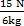= 2.5m/s2
Linear momentum = mass X velocity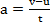2.5 =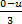U = 7.5
Linear momentum = 6kg x 7.5m/s
Linear momentum = 45kgm/s